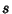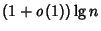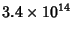## Rabin-Miller Strong Pseudoprime Test

A Primality Test which provides an efficient probabilistic Algorithm for determining if a given number is Prime. It is based on the properties of Strong Pseudoprimes. Given an Odd Integer, letwithOdd. Then choose a random integerwith. Iforfor some, thenpasses the test. A Prime will pass the test for all.

The test is very fast and requires no more thanmultiplications (mod), where Lg is the Logarithm base 2. Unfortunately, a number which passes the test is not necessarily Prime. Monier (1980) and Rabin (1980) have shown that a Composite Number passes the test for at most 1/4 of the possible bases.

The Rabin-Miller test (combined with a Lucas Pseudoprime test) is the Primality Test used by Mathematicaversions 2.2 and later (Wolfram Research, Champaign, IL). As of 1991, the combined test had been proven correct for all, but not beyond. The test potentially could therefore incorrectly identify a large Composite Number as Prime (but not vice versa). Strong Pseudoprime tests have been subsequently proved valid for every number up to.

References

Arnault, F. Rabin-Miller Primality Test: Composite Numbers Which Pass It.'' Math. Comput. 64, 355-361, 1995.

Miller, G. Riemann's Hypothesis and Tests for Primality.'' J. Comp. Syst. Sci. 13, 300-317, 1976.

Monier, L. Evaluation and Comparison of Two Efficient Probabilistic Primality Testing Algorithms.'' Theor. Comput. Sci. 12, 97-108, 1980.

Rabin, M. O. Probabilistic Algorithm for Testing Primality.'' J. Number Th. 12, 128-138, 1980.

Wagon, S. Mathematica in Action. New York: W. H. Freeman, pp. 15-17, 1991.